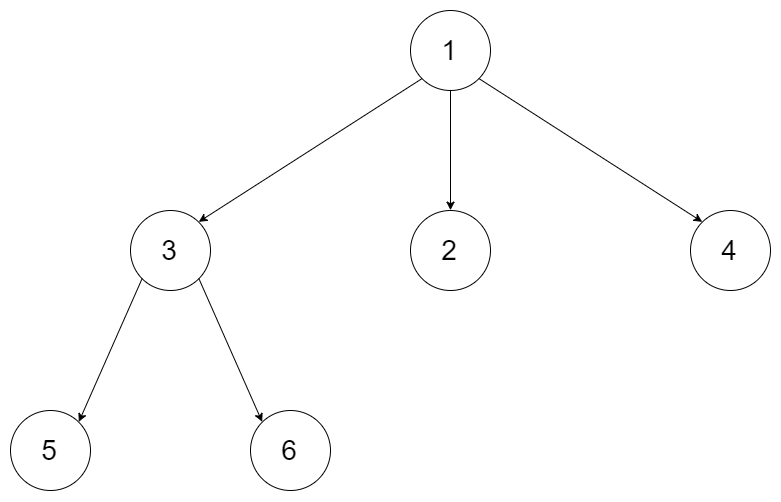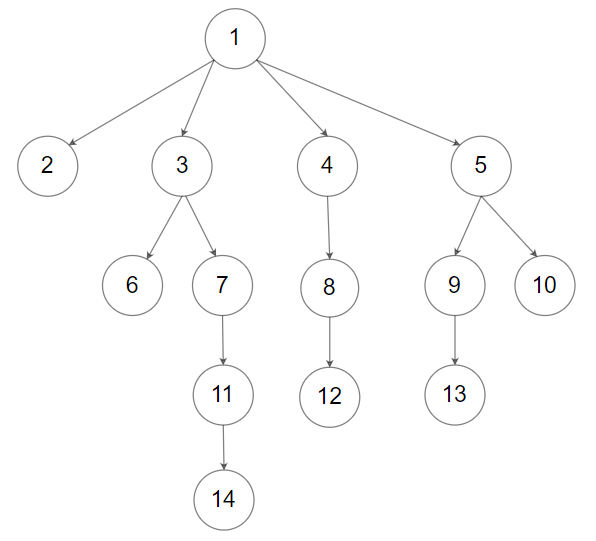##### Welcome to Subscribe On Youtube

Formatted question description: https://leetcode.ca/all/428.html

Hard

## Description

Serialization is the process of converting a data structure or object into a sequence of bits so that it can be stored in a file or memory buffer, or transmitted across a network connection link to be reconstructed later in the same or another computer environment.

Design an algorithm to serialize and deserialize an N-ary tree. An N-ary tree is a rooted tree in which each node has no more than N children. There is no restriction on how your serialization/deserialization algorithm should work. You just need to ensure that an N-ary tree can be serialized to a string and this string can be deserialized to the original tree structure.

For example, you may serialize the following 3-ary treeas [1 [3[5 6] 2 4]]. Note that this is just an example, you do not necessarily need to follow this format.

Or you can follow LeetCode’s level order traversal serialization format, where each group of children is separated by the null value.For example, the above tree may be serialized as [1,null,2,3,4,5,null,null,6,7,null,8,null,9,10,null,null,11,null,12,null,13,null,null,14].

You do not necessarily need to follow the above suggested formats, there are many more different formats that work so please be creative and come up with different approaches yourself.

Constraints:

• The height of the n-ary tree is less than or equal to 1000
• The total number of nodes is between [0, 10^4]
• Do not use class member/global/static variables to store states. Your encode and decode algorithms should be stateless.

## Solution

### 1). Serialization

If the tree is empty, return an empty string.

Do preorder traversal on the N-ary tree.

• Use a stack to store the nodes to be visited.
• Use parentheses to represent different levels and the children of each node.

### 2). Deserialization

If the serialization string is empty, return null.

Split the serialization string using ' '. Each term is a value of a node, a left parenthesis or a right parenthesis.

• Use two stacks to store the nodes and the split terms.
• Also use a map to store each node’s parent.

Loop over the split terms. If a left parenthesis is met, then the next term will be the previous node’s child. If a right parenthesis is met, then move to the parent of the current node. Otherwise, create a node using the current value. Finally, return the root.

• /*
// Definition for a Node.
class Node {
public int val;
public List<Node> children;

public Node() {}

public Node(int _val) {
val = _val;
}

public Node(int _val, List<Node> _children) {
val = _val;
children = _children;
}
};
*/
public class Serialize_and_Deserialize_N_ary_Tree {

class Codec {

private static final String SPLITER = ",";
private static final String NULL_NODE = "#";

// Encodes a tree to a single string.
public String serialize(Node root) {
StringBuilder sb = new StringBuilder();
buildString(root, sb);
return sb.toString();
}

// pre-order traversal
private void buildString(Node node, StringBuilder sb) {
if (node == null) {
sb.append(NULL_NODE).append(SPLITER).append(0).append(SPLITER); // size=0
} else {
sb.append(node.val).append(SPLITER).append(node.children.size()).append(SPLITER);
for (Node child: node.children) {
buildString(child, sb);
}
}
}

// Decodes your encoded data to tree.
public Node deserialize(String data) {
if (data.length() == 0) {
return null;
}

return buildTree(nodesList);
}

// @note: key is here, just keep popping the 1st as root of current sub-tree
String val = nodes.removeFirst();

if (val.equals(NULL_NODE)) {
return null;
} else {
Node node = new Node();
node.val = Integer.valueOf(val);
int size = Integer.valueOf(nodes.removeFirst()); // to get children size

node.children = new ArrayList<>(size);
for(int i = 0; i < size; ++i) {
}

return node;
}
}
}

// Your Codec object will be instantiated and called as such:
// Codec codec = new Codec();
// codec.deserialize(codec.serialize(root));

class Node {
public int val;
public List<Node> children;
public Node() {}
public Node(int _val, List<Node> _children) {
val = _val;
children = _children;
}
};

}

// Your Codec object will be instantiated and called as such:
// Codec codec = new Codec();
// codec.deserialize(codec.serialize(root));

• class Codec {
public:

// Encodes a tree to a single string.
string serialize(Node* root) {
string res;
serializeHelper(root, res);
return res;
}

void serializeHelper(Node* node, string& res) {
if (!node) res += "#";
else {
res += to_string(node->val) + " " + to_string(node->children.size()) + " ";
for (auto child : node->children) {
serializeHelper(child, res);
}
}
}

// Decodes your encoded data to tree.
Node* deserialize(string data) {
istringstream iss(data);
return deserializeHelper(iss);
}

Node* deserializeHelper(istringstream& iss) {
string val = "", size = "";
iss >> val;
if (val == "#") return NULL;
iss >> size;
Node *node = new Node(stoi(val), {});
for (int i = 0; i < stoi(size); ++i) {
node->children.push_back(deserializeHelper(iss));
}
return node;
}
};

• """
# Definition for a Node.
class Node(object):
def __init__(self, val, children):
self.val = val
self.children = children
"""
class Serialize_and_Deserialize_N_ary_Tree:

class Codec:

SPLITER = ","
NULL_NODE = "#"

# Encodes a tree to a single string.
def serialize(self, root: 'Node') -> str:
sb = []
self.buildString(root, sb)
return ''.join(sb)

# pre-order traversal
def buildString(self, node: 'Node', sb: List[str]):
if node is None:
sb.append(self.NULL_NODE + self.SPLITER + "0" + self.SPLITER)  # size=0
else:
sb.append(str(node.val) + self.SPLITER + str(len(node.children)) + self.SPLITER)
for child in node.children:
self.buildString(child, sb)

# Decodes your encoded data to tree.
def deserialize(self, data: str) -> 'Node':
if len(data) == 0:
return None

nodes_list = data.split(self.SPLITER)
return self.buildTree(nodes_list)

def buildTree(self, nodes: List[str]) -> 'Node':
# @note: key is here, just keep popping the 1st as root of current sub-tree
val = nodes.pop(0)

if val == self.NULL_NODE:
# pop the "0" added from sb.append(self.NULL_NODE + self.SPLITER + "0")
size = int(nodes.pop(0)) # here now size=0, but do nothing

# or, remove above 'size=...' line, but not adding "0" for null-node
# which is better I think

return None
else:
node = Node()
node.val = int(val)
size = int(nodes.pop(0))  # to get children size

node.children = []
for i in range(size):
node.children.append(self.buildTree(nodes))

return node

class Node:
def __init__(self, val=0, children=None):
self.val = val
self.children = children if children is not None else []

# Your Codec object will be instantiated and called as such:
# codec = Codec()
# codec.deserialize(codec.serialize(root))

###############

import struct
class Codec:
def _serialize(self, node):
self.data += struct.pack('i', node.val)
self.data += struct.pack('i', len(node.children))
for child in node.children:
self._serialize(child)
return

def serialize(self, root):
"""Encodes a tree to a single string.
:type root: Node
:rtype: str
"""
if root == None: return ""
self.data = ""
self._serialize(root)
return self.data

def _deserialize(self, data):
val = struct.unpack('i', data[self.idx:self.idx+4])
childrenNum = struct.unpack('i', data[self.idx+4:self.idx+8])
self.idx += 8
children = []
for i in range(childrenNum):
children.append(self._deserialize(data))
return Node(val, children)

def deserialize(self, data):
"""Decodes your encoded data to tree.
:type data: str
:rtype: Node
"""
if data == "": return None
self.idx = 0
return self._deserialize(data)

# Your Codec object will be instantiated and called as such:
# codec = Codec()
# codec.deserialize(codec.serialize(root))

`